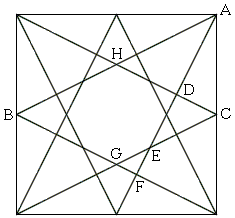Egyptian Triangle By Paper Folding

When the vertices of a square are joined to the midpoints of the nonadjacent sides -- eight lines in all, a starry shape is formed in which one may discern the famous 3:4:5 triangle, known as the Egyptian or the rope stretchers triangle.Thus the diagram provides an elegant way to form this triangle by paper folding.

In fact there are thirty two such triangles, eight of each type BFA, GFE, CDE, and HDA. A proof without words makes the assertion obvious for ΔBFA. The other triangles are clearly similar to the latter.

References

1. C. Alsina, R. B. Nelsen, Charming Proofs, MAA, 2010, p. 122
2. L. Bankoff, C. W. Trigg, The Ubiquitous 3:4:5 Triangle, Math Magazine, v 47, n 2 (Mar., 1974), pp. 61-70Paper Folding Geometry